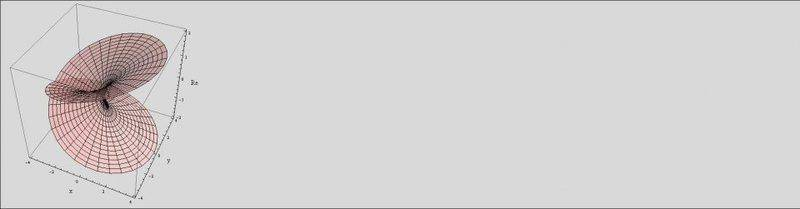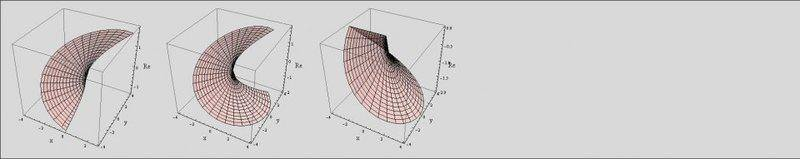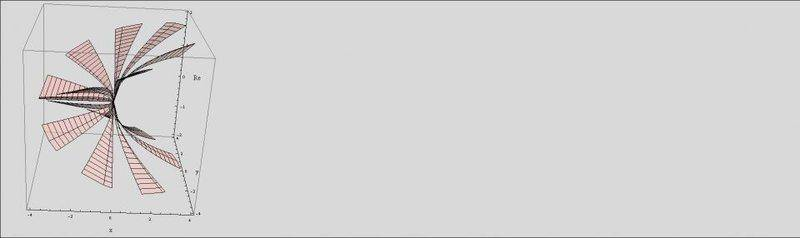# Branch points and branches

I have lately been trying to understand branch points and branches used for complex, multivalued, functions.

From doing questions and reading online, however, I get an unclear view of what branches points are.

Take the function: $$f(z)_a = (z^2+1)^\frac{1}{2}$$ or $$f(z)_b = log(z-1)$$

Based on my (limited) understanding of branch points, it seems that the points where the function is 0, f(1)_a and f(-1)_a, are branch points, or where it becomes undefined f(1)_b = log(0).

I do not understand why the function is 0, and sometimes undefined, at a branch point. I cannot find any text online that explains, clearly, exactly when you get a branch point.

Related to this of course is the notion of branches, which seem to be parts of the function, separated by a branch cut (a line between two branch points?).

Take for example, a more complicated example, $$f(z) = (z^2-i-1)^\frac{1}{4}$$

What method would I then use to find the branch points and the branches of this function?

I assume: $$z^2-i-1 = 0$$ Gives the two roots of z (two branch points), such that, generally, the nth root of z equals the branch points?

What about finding the branches, I assume that's related to the 1/4 exponential,but how do you explicitly go about doing it?

I have been trying to understand this for a few days, so any explanations would be really appreciated!

It's not correct that the branch points occur when the function is zero. You may have noticed it's related to when the arguments of radials or logs are zero. How does ##\ln |x| ## behave around ##|x| = 0## ?

The need for branch cuts has to do with the phase of the complex function. So, perhaps start with understanding the branch cuts and branch points for ##\sqrt{z}##.

WWGD
Gold Member
There are different types of branch points. In this case of ##z^2## , notice that if you wind around the origin once, you do not go back to the original
value, i.e., ## e^{i\theta/2} \neq e^{i(\theta+ 2\pi)/2}=-e^{i\theta}##. So you back to your original square root value after winding around twice. For ## z^n## you go back after
winding around n times, and for ##logz## , you never go back.

WWGD
Gold Member
The goal of the branch cut is to remove a part of the plane so that it is not possible for a curve to wind around the origin.

mathwonk
Homework Helper
branch points occur when we try to invert a function which is not injective. then we need to make a choice of which inverse image to take. this can occur fundamentally in two cases, (i) on a deleted neighborhood of a point where there is no inverse image, such as happens with log near zero, or (ii) on a deleted neighborhood of a point at which there is only one inverse image, as occurs with sort(z) near z = 0.

Hence it is clearer to examine a single valued function whose inverse is being used, than to puzzle over the multiple valued one. E.g. log should be viewed as the inverse of exp. since exp is many to one, to choose an inverse of it means choosing a subset on which it is injective. e.g. if U is a connected open set whose image under exp is simply connected, then exp is injective on U, and hence has an inverse defined on the image set exp(U). Equivalently, if V is a connected simply connected open subset of the punctured plane with 0 deleted, log can be defined on V, or as we say confusingly " a branch of the log exists on V". This use of the word branch differs from that denoting a branch point. The connection roughly speaking is that choosing a branch of the log is done by avoiding its branch point zero (on a set that does not wind around that branch point).

take one of your examples w = (z^2 + 1)^(1/2). Since this determines two values of w for most values of z, it is clearer to write instead w^2 = z^2 + 1, or the equation w^2 - z^2 - 1 = 0. Then look at this curve in the product of the complex plane with itself CxC. This defines a curve which does not pass the vertical line test, hence is not the graph of a single valued function. It is instead the graph of your multiple valued function.

Consider the projection from this curve to the z axis. This projection is usually 2 to 1, i.e. there are form most values of z, 2 values of w making a pair (z,w) on this graph. Any point of the graph where the projection is only one to one is called a ramification point on the graph, and its image value of z is called a branch point of the image set on the z axis. Near such a ramification point, the projection looks like the basic example z -- > z^2 near z=0.

Choosing a (single valued) "branch" of the function w(z) means choosing a subset of the z axis and a continuous inverse to the projection. One cannot do this on any neighborhood of a branch value of z. However it seems, at least intuitively, that one can do it on any connected simply connected open set of the z axis that does not contain any branch points.

by this definition notice that the map exp does not have any ramification points hence no branch values, but there is the point z = zero which is not a value at all, and it must be considered analogous to a branch point. i.e. before defining an inverse one removes all points which have no preImages (non values) as well as those which have too few (branch values).

in general, one looks at a (proper) map X-->Y, and excludes all branch values, so then one has a topological covering space, and one can then ask for local inverses to the restricted map, i.e. restricted to sets where it is injective. From Y, one excludes non values and branch values, then looks for a (right) inverse.

A branch cut, is usually a curve which contains all branch points and whose complement is connected and simply connected, so that there does exist an inverse on this complement. E.g. the non negative x axis in the complex plane is such a curve for exp, and there do exist many inverses of the exp map on its complement. For your last example, you could take any infinite ray containing both square roots of i+1. on the complement of this set there are 4 choices of inverses, i.e. of values of w.

to sum up, the way to picture a multivalued function w(z) is to look at its graph {(z,w): w = w(z)}, and at the projections from the graph to the two factor spaces, i.e. the z and w axes, and examine where the number of inverse images of the projections goes down. the good set (complement of the branch points) is the set where all points have the same number of inverse images.

Explicit inverses are not so easy to find. Even for simple polynomials the inverse will usually be a power series, but such series do exist at least on any disc contained in the complement of the set of non values and branch values. E.g. there are series for square roots and 4th roots of z^2+1 and z^2-i-1, for z near zero.

Last edited:
WWGD
Gold Member
Don't you also use branches to turn multi-functions into functions?

mathwonk
Homework Helper
yes if you mean branch cuts, i.e. the full inverse of a non injective function f is a multi valued function. the partial inverse of an injective restriction of f is a single valued function. such single valued functions can be found over sets which lie in the complement of appropriately chosen branch cuts. such a single valued partial inverse is also confusingly called a "branch" of the inverse.

But as to "branches" in the sense of branch points, - their role in turning multi functions into functions is that they should be avoided. i.e. to turn sort(z) into a function one avoids the branch point z = 0, by choosing a branch cut that includes z=0 and renders the plane simply connected when it is removed.

so the word branch has at least three different meanings: 1) (branch point) a point where a function has fewer than the usual number of inverse images, 2) (branch cut) a cut that eliminates such points, and 3) (single valued branch) a single valued inverse defined on the complement of such a cut. very confusing.

f(z)a=(z2+1)12
f(z)_a = (z^2+1)^\frac{1}{2} or
f(z)b=log(z−1)
f(z)_b = log(z-1)
1. I think, this is not correct notation. If you denote your function with $f_{\mathrm a}$ and $f_{\mathrm b}$, then, the correct call of these function with argument $z$ should have forms $f_{\mathrm a}(z)$ and $f_{\mathrm b}(z)$, but not $f(z)_a$ nor $f(z)_b$.

2. Now I have similar problem. I deal with polynomial $T(z)=z+z^3+qz^4$ where $q$ is positive parameter. It happens to be not so easy to construct function $T^{-1}$ with cuts along the lines I choose. Well, I have constructed one example for $q=1$, the complex map is at http://mizugadro.mydns.jp/t/index.php/File:Aunem11mbp.jpg
but I dislike the way I do it. I believe, the same can be done in a more civilised mode. I should appreciate any hint on this.

lavinia
Gold Member
Don't you also use branches to turn multi-functions into functions?

Also one can turn a multi-valued function on the complex plane into a single value function on its Riemann surface.

I've done a lot of work with multi-valued functions and in my humble opinion there is no better way to cultivate a basic (non-Riemann surface) intuitive understanding of branches, branch-cuts, and branch-points by simply plotting the imaginary and real components of some simple algebraic functions and working with their multi-valued surfaces. Here's one I've done some work with:

##\displaystyle\int_C z^{1/3}(1-z)^{1/2} dz##

Now plot it, integrate it, tear it apart, cut it, paste it, and excise the (analytically-continuous) branching geometry around the (3) singular points. Do that for a few weeks and I guarantee you'll come to a good understanding of branch-points and branch-cuts.

Edit: Oh wait, I'm sorry, I mean do this one first:

##\displaystyle\int_C z^{1/2}dz##

and then maybe a few more a little more complicated than that, then do that other one. Here's the analytically-continuous real surface of the square-root function:Last edited:
mathwonk
Homework Helper
In reference to Lavinia's remark, in most cases the Riemann surface actually equals the graph. I.e. in the example w = sqrt(z), a 2 valued function of z, one has as graph the curve w^2 = z in the product space CxC. Although the assignment taking z to w = sqrt(z), is 2 valued for all z ≠ 0, if instead of the z axis, we choose the graph itself as the domain of our function, then the projection mapping taking a point (z,w) of this graph to w, will be a single valued version of that function. I.e. the graph of all pairs (z,w) such that w^2 = z, is essentially the Riemann surface of the 2 valued function w = sqrt(z). The function becomes singlke valued there precisely because giving a pair (z,w) already picks out a specific square root w of z. I.e. there are two pairs (z,w) on thw graph for each value of z, hence we have doubled the number of points in the domain and the 2 valued function becomes single valued. In general that is what the Riemann surface does, it provides a larger domain, so that if a multivalued function originally was say 4 valued, the Riemann surface will have 4 points for each point of the origional domain, allowing each value to be taken on one of the new domain points. In general, except for singularities and points at infinity, the Riemann surface of a multivalued function w = f(z), is just the graph consisting of all pairs (z,w) satisfying w = f(z). I.e. even if w is a multiple valued function of z, it is a single valued function of (z,w). More precisely, the true Riemann surface is obtained from this graph by separating singularities (points where the graph crosses itself or is "kinked"), and adding in missing points "at infinity".

Last edited:
mathwonk
Homework Helper
here are some notes for the 1st 3 days of a course on Riemann surfaces.

#### Attachments

• 8320sp2010.day1-3.pdf
107 KB · Views: 383
FactChecker
Gold Member
The idea behind a branch point zb of the function f is this: You can start with z at a point, z0 on a small circle around zb. As you proceed around the circle, the function, f(z) is very nice, smooth, and has all derivatives,(it's analytic). But when you get back to z0, the continuous value of f(z0) is not the same as when you started. There is no valid reason to prefer one value of f(z0) over another. So we say that going around zb has actually taken us to a different point than when we started. We are at a different place on a Riemann surface. The actual value of f at zb is not important. It might not even be defined.

WWGD
Gold Member
I think in a very general sense, a branch point is a type of singularity and a branch cut is a removal of a subset of the Complex plane done to avoid having any curves winding around the branch point(s), since it is this--winding around the branch point(s) that makes a function into a multifunction ; I think this is a generalization of the result that for f(z) analytic in a region, a holomorphic logf(z) exists iff ## f\neq 0 ## and ##f(z)## does not wind around the origin. I will try to expand on this soon.

I wish to continue with my elementary treatment of the subject using the canonical square-root function: We're concerned in Complex Analysis with analyticity so that we may use theorems such as Laurent's Theorem, the Residue Theorem, Cauchy's Theorems, and others. So naturally we wish to identify non-analytic conditions of multivalued functions. Branch-points are such non-analytic conditions, where the function cease to be (locally) analytic. In the case of ##f(z)=\sqrt{z}##, obviously the function is non-analytic at the origin, it's derivative becoming undefined there. The function is however (locally) analytic everywhere else in the finite complex plane although it is not single-valued and in order to use the theorems above and the rest of basic Complex Analysis, we require single-valued functions. But we can excise single-valued portions of the plot and those pieces are called "single-valued branches" of the function and will be applicable to the entire foundation of Complex Analysis of single-valued analytic functions. The excisions are purely arbitrary; just any single-valued piece would do. For example, I can (arbitrarily excise say the portion running from ##(9\pi/4, 14\pi/4)##. That section is a (single-valued) branch of the function, the real part shown in the first plot. Generally though, we wish to cut out the maximum section that remains single-valued. That too is easy, just choose a radial line, and cut out a section right before it begins to overlap. In the second plot, I've cut out a maximum section along the line ##5\pi/8##. That's another single-valued branch segment. Usually though we just cut it along the negative real axis which is shown in the third plot. This being a third single-valued branch section.

And that's basically it for branch-points, branch cuts and (single-valued) branches.

So my suggestion for any student reading this and planning to take Complex Analysis this fall is to work with these examples, learn how to plot them in Mathematica, do what I suggested above with them so by the time September rolls around, you'll be ready for what often is the most difficult concept to master in Complex Analysis and a cause for many to drop the class.Last edited:
Here's the real part of an unusual (single-valued) branch of the square root function where I've excised single-valued ##\pi/8## sections around the multivalued sheet (if you looked at it from the top, there would be no overlapping sections and of course the imaginary component would be likewise so):This plot I think shows dramatically, that the whole purpose of branch-cutting is simply to excise a single-valued section of a multi-valued (overlapping) sheet so that we can then apply all the complex-analysis theorems of single-valued functions to multi-valued functions prior to learning about Riemann surfaces which is a bit more advanced for a basic Complex Analysis course.

Last edited:
WWGD
Gold Member
Another relatively-straightforward example is that of the complex log, logz. Here you can also use the inverse function thm, given that ##d/dzlogz=1/z ## (this is true only within a branch, though, so maybe somewhat circular) the issue is that ##e^z##, its local inverse, is ##\infty##-to-1, by periodicity of trigonometric functions. Since the function is ## 2\pi## -periodic, you must restrict yourself to vertical strips of height ##2\pi^{-}## .

mathwonk